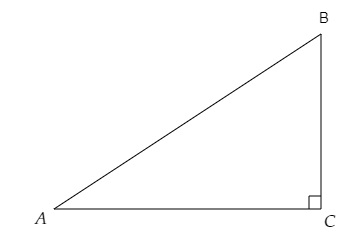# If $\angle A$ and $\angle B$ are acute angles such that $cos\ A = cos\ B$, then show that $‚ą†A = ‚ą†B$.

Given:

$\angle A$ and $\angle B$ are acute angles such that $\cos A=\cos B$.

To do:

We have to show that $\angle A=\angle B$.

Solution:

Let, in a triangle $ABC$ right angled at $C$, $cos\ A = cos\ B$.We know that,

In a right-angled triangle $ABC$ with a right angle at $C$,

By trigonometric ratios definitions,

$cos\ A=\frac{Adjacent}{Hypotenuse}=\frac{AC}{AB}$

$cos\ B=\frac{Adjacent}{Hypotenuse}=\frac{BC}{AB}$

This implies,

$\cos A=\cos B$

$\Rightarrow \frac{AC}{AB}=\frac{BC}{AB}$

$\Rightarrow AC=BC$

We know that,

Angles opposite to equal sides are equal in a triangle.

Therefore,

$\angle A=\angle B$

Hence proved.

Updated on: 10-Oct-2022

62 Views